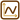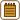## Reflection of Light in a Glass Horseshoe

### Task number: 2214

A glass rod of rectangular section is bent into a shape of a „horseshoe“ (see picture). The inner bend of the horseshoe is circular with radius $$R$$ and the outer bend is also circular with radius $$R+d$$.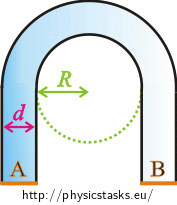A bundle of parallel light rays is incident perpendicularly on area A. Determine the minimal value of the fraction $$\frac{R}{d}$$ so that all the light from this bundle leaves the horseshoe through area B.

• #### Hint 1

Because we want all the rays to go through the area B they must be totally reflected in the horseshoe. Think of when the total inner reflection occurs and write Snell’s law for the total inner reflection of light.

• #### Hint 2

Think about if it is necessary to deal with all the light rays from the bundle.

• #### Hint 3

We are now going to study the path of a ray in the „bent“ part of the horseshoe.

Imagine two concentric circles with a glass annulus between them. Then imagine a ray whose trajectory is a tangent to the „inner“ circle and which is incident on the outer circle at an angle $$α$$.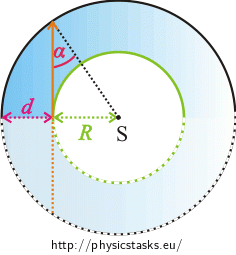How will the ray continue? Draw it in the picture.

• #### Hint 4

At which angle does the ray hit point M?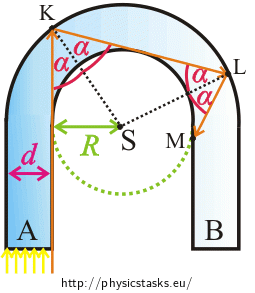Will the ray be totally reflected in the point M? Draw the trajectory of the ray in the rest of the horseshoe in the picture.

• #### Hint 5

We found out that if the ray hits the point K at an angle $$α \gt α_{m}$$, it is being totally reflected until it leaves the horseshoe.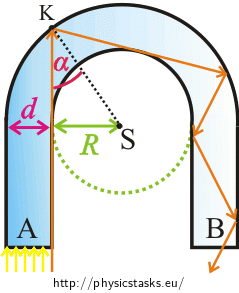Use the picture to determine the relation between the angle $$α$$, radius $$R$$ and the width of the horseshoe $$d$$. Then use relation (2) to determine the value of the fraction $$\frac{R}{d}$$.

• #### Complete solution

Total internal reflection

Because we want all the rays to go through area B they must be totally reflected in the horseshoe.

For total inner reflection to occur, the angle of incidence $$α$$ of the ray must be greater than the critical angle $$α_{m}$$: $α \gt α_{m}.$ If the angle of incidence is less than $$90°$$ while it holds that $$α \gt α_{m}$$ it also holds that: $\sin{α} \gt \sin{α_{m}}.\tag{1}$

Critical angle is such an angle when the ray is still refracted. The angle of refraction is $$90°$$. In this case we write Snell’s law as: $\frac{\sin{α_{m}}}{\sin{90°}}=\frac{n_{a}}{n_{g}},$

where $$n_{a}$$ is the index of refraction of the air and $$n_{g}$$ is the index of refraction of glass in which the light rays propagate.

It holds that $$\sin{90°}=1$$ and from the tables we know that $$n_{a} \dot= 1$$. Thus: $\sin{α_{m}}=\frac{1}{n_{g}}.$ We substitute this relation into relation (1): $\sin{α} \gt \frac{1}{n_{g}}.\tag{2}$

One ray is enough

We do not have to care about all the rays from the light bundle. It is sufficient to work with the extreme (orange) ray. If the orange ray is totally reflected every other ray is totally reflected as well (other rays hit the outer circle at a greater angle than is the angle of incidence of the orange ray).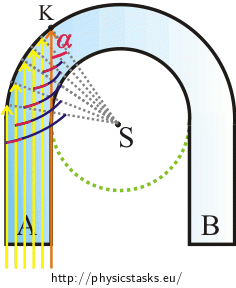Thus, if the orange ray is totally reflected when it hits the glass-air boundary for the first time, it is totally reflected in the whole horseshoe. (See Hint 3 a Hint 4.)

Minimal value of the fraction $$\frac{R}{d}$$

If we look closely at the right-angled triangle in the picture, we find out that the length of its hypothenuse is equal to the sum $$R+d$$.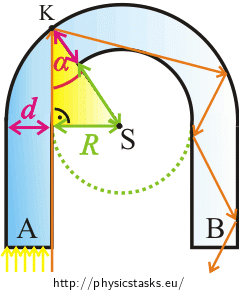Using the sine function, we get: $\sin{α}=\frac{R}{R+d}.\tag{3}$ Remember relation (2): $\sin{α} \gt \frac{1}{n_{g}}.$ $\frac{R}{R+d}\gt \frac{1}{n_{g}} .$ As we are also interested in the limit case (when the angle of incidence on the outer circle $$α$$ is equal to the critical angle $$α_{m}$$ and thus also $$\sin{α}=\sin{α_{m}}$$), we will write this inequality as: $\frac{R}{R+d}\ge \frac{1}{n_{g}} .$ We rewrite the inequality for clarity: $\frac{1}{n_{g}} \le \frac{R}{R+d}.$ We multiply both sides of the inequality by positive expression $$\frac{R+d}{R}$$: $\frac{1}{n_{g}}\frac{R+d}{R} \le 1.$ We simplify the inequality to get $$\frac{R}{d}$$ on the left side: $\frac{1}{n_{g}}\left(\frac{R}{R}+\frac{d}{R} \right)\le 1,$ $\frac{1}{n_{g}}\left(1+\frac{d}{R} \right)\le 1,$ $\frac{1}{n_{g}}+\frac{1}{n_{g}}\frac{d}{R} \le 1,$ $\frac{1}{n_{g}}\frac{d}{R} \le 1 − \frac{1}{n_{g}},$ $\frac{1}{n_{g}}\frac{d}{R} \le \frac{n_{g}−1}{n_{g}},$ $\frac{d}{R} \le n_{g}−1,$ $\frac{R}{d} \ge \frac{1}{ n_{g}−1}.$

Numerical solution

We find the value of refraction index of glass in tables $$n_{g} = 1{,}5$$ and substitute it into equation: $\frac{R}{d} \ge \frac{1}{ 1{,}5−1},$ $\frac{R}{d} \ge 2.$

The minimal value of the fraction $$\frac{R}{d}$$ must be equal to two for the total internal reflection to occur in the horseshoe.